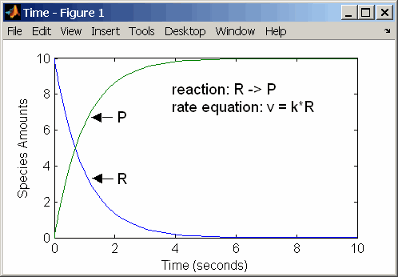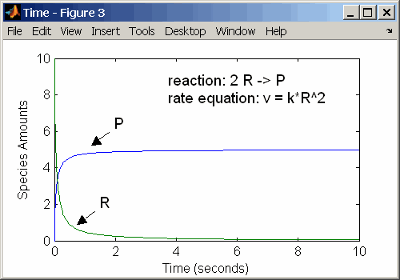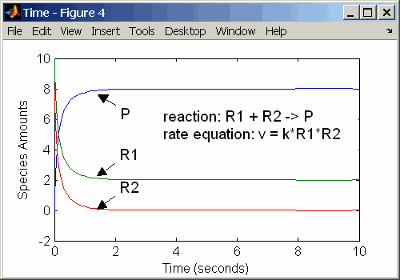## Define Reaction Rates with Mass Action Kinetics

Use mass action kinetics to define zero-order, first-order, second-order, and reversible reactions.

### Definition of Mass Action Kinetics

Mass action describes the behavior of reactants and products in an elementary chemical reaction. Mass action kinetics describes this behavior as an equation where the velocity or rate of a chemical reaction is directly proportional to the concentration of the reactants.

### Zero-Order Reactions

With a zero-order reaction, the reaction rate does not depend on the concentration of reactants. Examples of zero-order reactions are synthesis from a `null` species, and modeling a source species that is added to the system at a specified rate.

``` reaction: null -> P reaction rate: k mole/second species: P = 0 mole parameters: k = 1 mole/second```

Note

When specifying a `null` species, the reaction rate must be defined in units of amount per unit time not concentration per unit time.

Entering the reaction above into the software and simulating produces the following result:

Zero-Order Mass Action KineticsNote

If the amount of a reactant with zero-order kinetics reaches zero before the end of a simulation, then the amount of reactant can go below zero regardless of the solver or tolerances you set.

### First-Order Reactions

With a first-order reaction, the reaction rate is proportional to the concentration of a single reactant. An example of a first-order reaction is radioactive decay.

``` reaction: R -> P reaction rate: k*R mole/(liter*second) species: R = 10 mole/liter P = 0 mole/liter parameters: k = 1 1/second ```

Entering the reaction above into the software and simulating produces the following results:

First-Order Mass Action Kinetics### Second-Order Reactions

A second-order reaction has a reaction rate that is proportional to the square or the concentration of a single reactant or proportional to two reactants. Notice the space between the reactant coefficient and the name of the reactant. Without the space, `2R` would be considered the name of a species.

``` reaction: 2 R -> P reaction rate: k*R^2 mole/(liter*second) species: R = 10 mole/liter P = 0 mole/liter parameters: k = 1 liter/(mole*second) ```

Entering the reaction above into the software and simulating produces the following results:

Second-Order Kinetics with Single ReactantWith two reactants, the reaction rate depends on the concentration of two of the reactants.

``` reaction: R1 + R2 -> P reaction rate: k*R1*R2 mole/(liter*second) species: R1 = 10 mole/liter R2 = 8 mole/liter P = 0 mole/liter parameters: k = 1 liter/(mole*second) ```

Enter the reaction above into the software and simulating produces the following results. There is a difference in the final values because the initial amount of one of the reactants is lower than the other. After the first reactant is used up, the reaction stops.

Second-Order Kinetics with Two Reactants### Reversible Mass Action

You can model reversible reactions with two separate reactions or with one reaction. With a single reversible reaction, the reaction rates for the forward and reverse reactions are combined into one expression. Notice the angle brackets before and after the hyphen to represent a reversible reaction.

``` reaction: R <-> P reaction rate: kf*R - kr*P mole/(liter*second) species: R = 10 mole/liter P = 0 mole/liter parameters: kf = 1 1/second kr = 0.2 1/second ```

Entering the reaction above into the software and simulating produces the following results. At equilibrium when the rate of the forward reaction equals the reverse reaction, ```v = kf*R - kr*P = 0``` and `P/R = kf/kr`.## SupportGet trial now